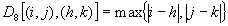The digitized image and its properties

Image functions

• An image may be modeled as a continuous function of 2 variables [coordinates (x,y) in the plane] or 3 variables [when the time t is added]. Image processing often deals with static images (constant t).
• Image function values correspond to the brightness at image points and the image is thus called intensity image.
• The image on the human retina or on a TV camera is intrinsically 2D. Few real world applications are 2D such as character images, fingerprints, or images under a microscope. The real world, on the other hand, is intrinsically 3D.
• The 2D image is created as a result of perspective projection of the 3D scene, modeled by the image captured by a pin-hole camera.
• A projected point (x, y, z) has coordinates (x’, y’) in the 2D image plane where: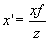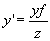where f is the focal length of the camera.

• A lot of information is lost in this transformation since it is not one-to-one. Reconstructing the 3D image from the projected 2D image is an ill-posed problem.
• The brightness of a pixel depends on several independent factors:
1. Object surface reflectance.
2. Illumination properties.
3. Object surface orientation with respect to a viewer and the light source.
• Separating the components of brightness is another ill-posed problem.

• The domain of an image function is a region R in the plane: R = { (x, y), 1 £ x £ xm, 1 £ y £ yn }
• The range of image function is also limited. In monochromatic images, the lowest value corresponds to black while the highest value corresponds to white. Brightness values bounded by that range are called graylevels.
• In general, functions may be categorized into:

 Type Domain Range Continuous Continuous Continuous Discrete Discrete Continuous Digital Discrete Discrete
• The quality of the digital image grows in proportion to the image resolution:
1. Spatial resolution: given by the proximity of the image samples in the image plane.
2. Spectral resolution: given by the bandwidth of light frequencies captured by the sensor.

3. Radiometric resolution: corresponds to the number of distinguishable graylevels.
4. Time resolution: given by the interval between time samples at which images are captured.

Image digitization

• Image digitization means that a continuous function f(x,y) is sampled into a matrix with M rows and N columns.
• Image quantization assigns to each continuous sample an integer value.
• Two questions should be answered in connection with image function sampling:
1. The sampling period:
• Based on Shannon sampling theorem. The sampling interval should be chosen in size such that it is less than half of the smallest interesting detail in the image.
• Decreasing the sampling period degrades the image quality. Much of the degradation is caused by aliasing in the reconstruction of the continuous image function for display. The display can be improved by the reconstruction algorithm interpolating brightness values in neighboring pixels.
1. The geometric arrangements of the sample points:
• Sampling points are ordered on a grid in the plane.
• Grids used in practice are usually square (rectangular) or hexagonal. Unless otherwise mentioned, we will always use the rectangular sampling grid.

• Each sampling point on the grid is called a pixel.

Quantization

• The transition between continuous values of the image brightness and its digital equivalent.
• The number of quantization levels should be high enough to permit human perception of the shading details of the image.
• Most image processing devices perform quantization into k equal intervals (usually k=256 levels).
• The number of bits required to represent k values is b = log2k. (k=256 needs 8 bits per pixel).
• A binary image pixel can be represented by one bit (black or white).
• Devices using 12 bits per pixel (k=4096) are becoming more common.
• An image quantized with lower brightness levels than that which humans can easily distinguish (at least 100) will suffer from false contours.

Color (multi-spectral) images

• Color images are becoming more popular with the availability of cheaper memory and hardware.
• Color is connected with the ability of objects to reflect electromagnetic waves of different wavelengths. (chromatic spectrum ranges from 400 to 700 nm).
• Color may be generated by combining the 3 primary colors: red (700 nm), green (546.1 nm) and blue (435.8 nm). This is called the RGB model.
• Each pixel has a 3 dimensional vector (r, g, b) associated with it. Example colors include: (0, 0, 0) BLACK, (k, k, k) WHITE, (k, 0, 0) RED, (0, K, 0) GREEN, (0, 0, k) BLUE.
• For k = 256, the number of distinct colors is: 256 x 256 x 256 = 224.
• The secondary colors are created as combination of the primary colors: (cyan = green + blue, magenta = red + blue, yellow = red + green).
• The CMY – Cyan, Magenta, Yellow – is based on the secondary colors and is used to construct a subtractive color scheme used for combining inks in color printers. e.g. yellow subtracts blue from white while a combination of yellow and magenta subtracts green and blue from white giving red.
• The YIQ model is useful for TV broadcasting and it is created from RGB as follows: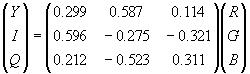• The HSI – Hue, Saturation, Intensity – is the most relevant to image processing. Hue refers to the perceived color or the dominant wavelength and saturation measures the dilution of the color by white light (dark or light).
1. Decouples the intensity information from color information.
2. Hue and saturation correspond to human perception.
• To convert from RGB to HSI representation: normalize rgb colors so that 0 £ r, g, b £ 1. Then,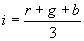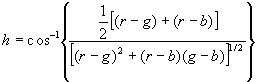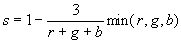If b/i > g/i. set h=2p -h.

• These measurements are normalized to the range [0,1] if h is set to h/2p .
• If r=g=b, h is undefined and if i=0, s is undefined.
• To convert back from HIS to RGB, there are 3 cases to consider. Writing H=2p h.

Case 0 < H £ 2p /3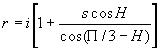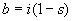Case 2p /3 < H £ 2 x 2p /3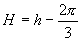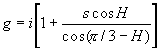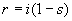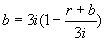Case 2 x 2p /3 < H £ 2p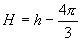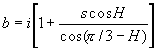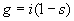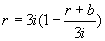Digital image properties

Distance

• Euclidean distance DE :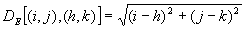• intuitive distance calculation

• costly calculation due to square root
• result is a non-integer value
• City block distance (D4 distance): The minimum number of steps on the grid needed to move from a starting point to an end point. Only horizontal and vertical moves are allowed.• Chessboard distance (D8 distance): The minimum number of steps on the grid needed to move from a starting point to an end point. Horizontal, vertical and diagonal moves are allowed (like the king of chess).